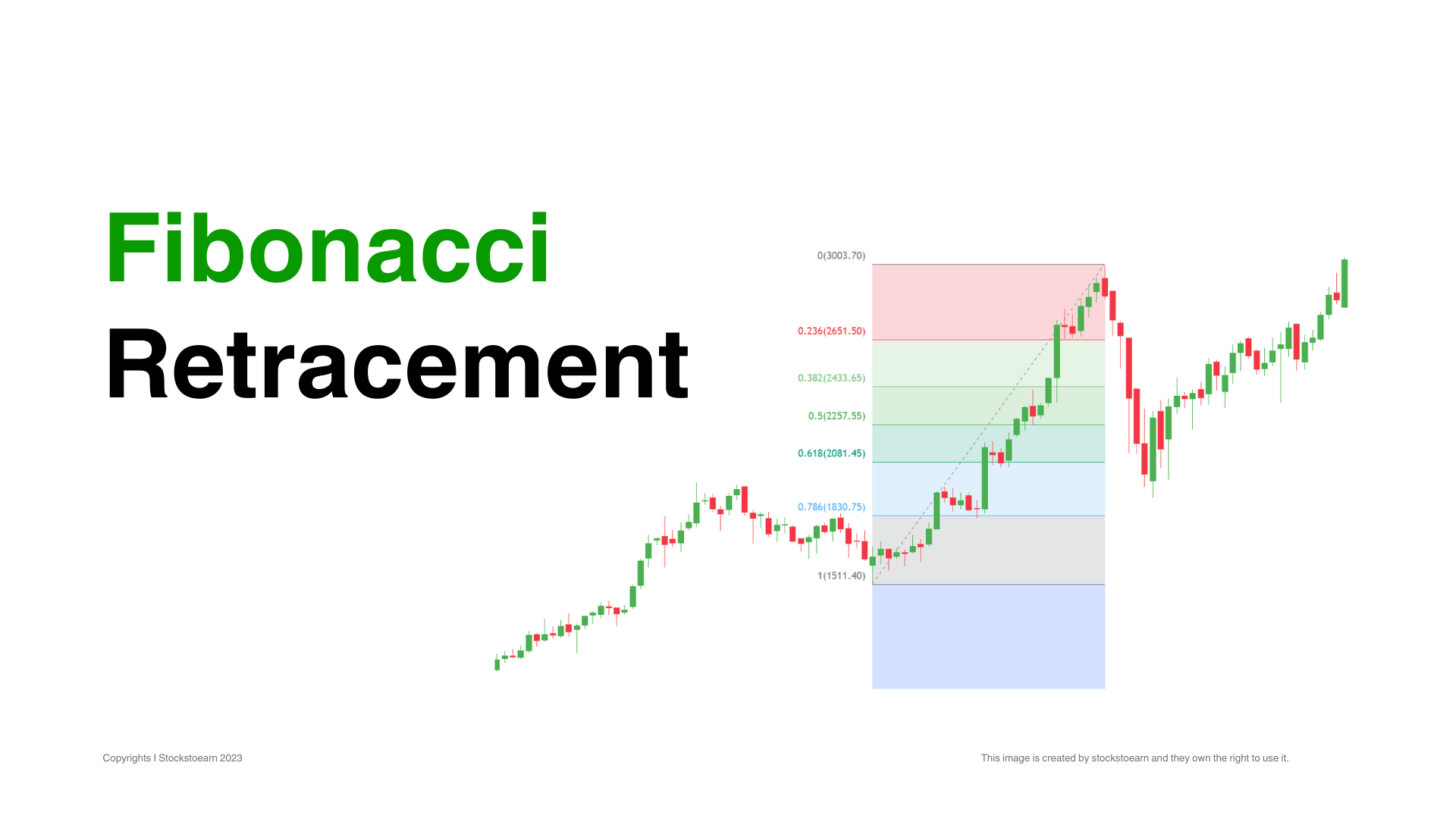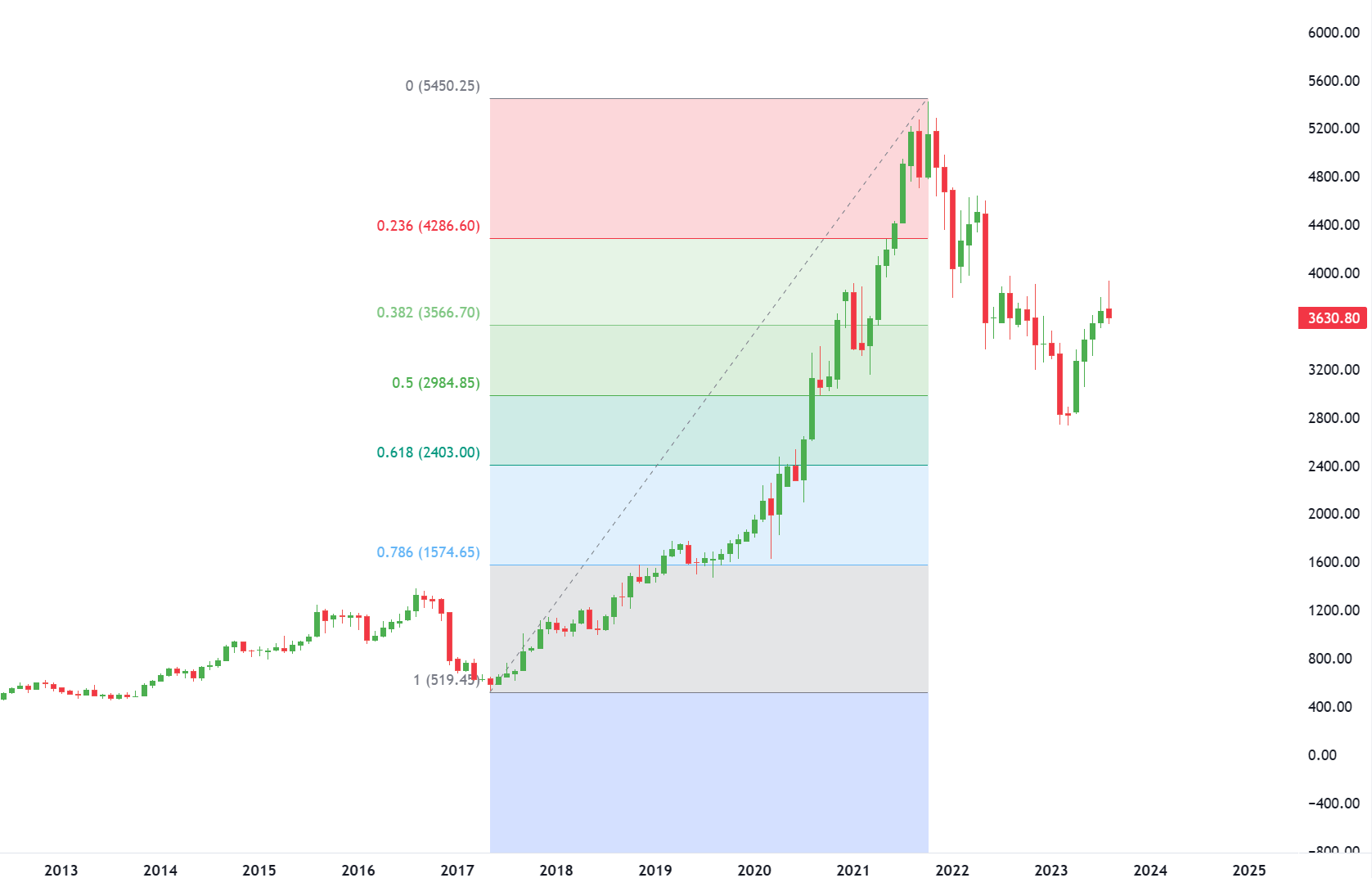### Fibonacci RetracementFibonacci retracement levels are based on ratios used to identify potential reversal points on a price chart. These ratios are found in the Fibonacci sequence. The most popular Fibonacci retracements are 61.8% and 38.2%. Note that 38.2% is often rounded to 38%, and 61.8 is rounded to 62%.

#### Learning Tip

After an advance, chartists apply Fibonacci ratios to define retracement levels and forecast the extent of a correction or pullback. Fibonacci retracement levels can also be applied after a decline to forecast the length of a counter-trend bounce. These retracements can be combined with other indicators and price patterns to create an overall strategy.

Crucial Levels

#### Fibonacci Levels as Alert Zones

Retracement levels alert traders or investors of a potential trend reversal, resistance area or support area. Retracements are based on the prior move. A bounce is expected to retrace a portion of the prior decline, while a correction is expected to retrace a portion of the prior advance.

Once a pullback starts, chartists can identify specific Fibonacci retracement levels for monitoring. As the correction approaches these retracements, chartists should become more alert for a potential bullish reversal.

Keep in mind that these retracement levels are not hard reversal points. Instead, they serve as alert zones for a potential reversal. It is at this point that traders should employ other aspects of technical analysis to identify or confirm a reversal. These may include candlesticks, price patterns, momentum oscillators or moving averages.

Classification

#### Types of Retracement LevelsThe Fibonacci Retracements shows four common retracements: 23.6%, 38.2%, 50%, and 61.8%. From the Fibonacci section above, it is clear that 23.6%, 38.2%, and 61.8% stem from ratios found within the Fibonacci sequence. The 50% retracement is not based on a Fibonacci number. Instead, this number stems from Dow Theory’s assertion that the Averages often retrace half their prior move.

Based on depth, we can consider a 23.6% retracement to be relatively shallow. Such retracements would be appropriate for flags or short pullbacks. Retracements in the 38.2%-50% range would be considered moderate. Even though deeper, the 61.8% retracement can be called golden retracement. It is, after all, based on the Golden Ratio.

Fibonacci retracement levels are often used to identify the end of a correction or a counter-trend bounce. Corrections and countertrend bounces often retrace a portion of the prior move.

Fibonacci retracements can be combined with other indicators such as candlesticks, price patterns, momentum oscillators, or moving averages to create a robust trading strategy and confirm potential reversals.